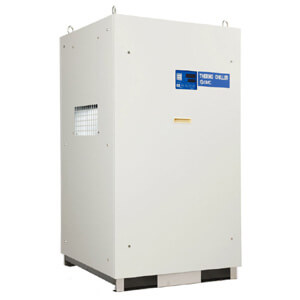# HRSH, Large Capacity, High Efficiency Inverter Chiller, Water-cooled 200VAC

HRSH Water-cooled recirculating chillers offer a cooling capacity range of 11.5 to 24 kW. Coolant and refrigeration circuits are included in a compact and lightweight package. Extracted heat is water cooled. An inverter provides demand-based rotation control for the compressor, pump and fan (air cooled model), offering substantial energy savings. Electric options ensure global power supply compatibility. Additional features include a heating function, a low-maintenance seal-less immersion pump, and IPX4 rating for outdoor installation.

• Thermo-chiller, Large Type, Water-cooled 200 V Type.
• Cooling capacity: 11.5 kW, 15.7 kW, 20.6 kW, 24 kW.
• Temperature range setting: 5 to 35° C
• Temperature stability: ±0.1° C
• Outstanding energy saving effect with the triple inverter. Power consumption reduced by 53 %.
• Compact, Space-saving.Configuration OptionsCopy Configuration Number

## Product Options

Select Product Options
 Select values from the drop down lists or make appropriate selections to configure the part number. Cooling Capacity -- Pick an Option -- 100 (11.5kW) 150 (15.7kW) 200 (20.5kW) 250 (25kW)Thread -- Pick an Option -- Rc F (G, w/Rc-G Conversion Fitting) N (NPT, w/Rc-NPT Conversion Fitting)Option -- Pick an Option -- None A (w/Caster Adjuster-foot) AB (A + B) ABK (A + B + K) ABKM (A+ B + K + M) ABKMT (A+ B + K + M + T) ABKMTW (A+ B + K + M + T + W) ABKMW (A + B + K + M + W) ABKT (A + B + K + T) ABKTW (A + B + K + T + W) ABKW (A + B + K + W) ABM (A + B + M) ABMT (A + B + M + T) ABMTW (A + B + M +T + W) ABT (A + B + T) ABW (A + B + W) AB1 (A + B1) AB1K (A + B1 + K) AB1KM (A + B1 + K + M) AB1KMT (A + B1 + K + M + T) AB1KMTW (A + B1 + K + M + T + W) AB1KMW (A + B1 + K + M + W) AB1KT (A + B1 + K + T) AB1KTW (A + B1 + K + T + W) AB1KW (A + B1 + K + W) AB1T (A + B1 + T) AB1MT (A + B1 + M + T) AB1MTW (A + B1 + M + T + W) AB1TW (A + B1 + T + W) AB1W (A + B1 + W) AK (A + K) AKMS (A + K + M + S) AKMST (A + K + M + S + T) AKMSTW (A + K + M + S + T + W) AKS (A + K + S) AKSW (A + K + S + W) AKT (A + K + T) AKW (A + K + W) AM ( A + M) AMS (A + M + S) AMST (A + M + S + T) AMSTW (A + M + S + T + W) AMSW (A + M + S + W) AMT (A + M + T) AMW (A + M + W) AS (A + S) AST (A + S + T) ASW (A + S + W) AT (A + T) ATW (A + T + W) AW (A + W) B (w/Earth Leakage Breaker) BK (B+K) BKT (B + K + T) BKTW (B + K + T + W) BKW (B + K + W) BM (B + M) BMT (B + M + T) BMTW (B + M + T + W) BT (B + T) BTW (B + T + W) B1 (w/Earth Leakage Braker w/Handle) B1K (B1 + K) B1KT (B1 + K + T) B1KTW (B1 + K + T + W) B1KW (B1 + K + W) B1MT (B1 + M + T) B1MTW (B1 + M + T + W) B1TW (B1 + T + W) B1W (B1 + W) BW (B + W) K (w/Fluid Fill Port) KM (K + M) KMS (K + M + S) KMST (K + M + S + T) KMSTW (K + M + S + T + W) KMT (K + M + T) KMTW (K + M + T + W) KMW (K + M + W) KS (K + S) KST (K + S + T) KSTW (K + S + T + W) KSW (K + S + W) KT (K + T) KW (K + W) M (Applicable to DI Water Piping) MS (M + S) MST (M + S + T) MSTW (M + S + T + W) S (Conforming to CE/UL Standards) ST (S + T) STW (S + T + W) SW (S + W) T (High-pressure Pump Mounted) W [SI Unit Only (Not Available in Japan Due to Measurement Law)]Options in: Gray are not compatible with other selected option value. Red conflict with another selected option value. Amber are not recommended.

Model does not exist, please email cad@smcusa.com with the complete part number, CAD file format and version required.